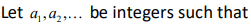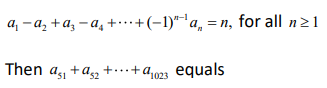### CAT 2019 – Slot 1 – Quantitative Ability – In a six-digit number, the sixth, that is, the rightmost, digit is the sum of the first three digits

Q. 1: In a six-digit number, the sixth, that is, the rightmost, digit is the sum of the first three digits, the fifth digit is the sum of first two digits, the third digit is equal to the first digit, the second digit is twice the first digit and the fourth digit is the sum of fifth and sixth digits. Then, the largest possible value of the fourth digit is

the smallest possible value of first digit = 1

So 3rd digit = 1, 2nd digit = 1*2 = 2

5th digit = 1+2= 3

6th digit = 1+2+1 =4

4th digit = 3+4 =7

When value of first digit = 2

So 3rd digit = 2, 2nd digit = 2*2 = 4

5th digit = 1+4= 5

6th digit = 2+2+4=8

4th digit = som of 5th and 6th digit = 5+8 =13 (not possible )

##### Q. 2: The quadratic equation x^2 + bx + c = 0 has two roots 4a and 3a, where a is an integer. Which of the following is a possible value of b^2 + c?1. 37212. 5493. 3614. 427

Sum of roots = -b

4a + 3a = -b

b^2 = 49a^2

similarly product of roots = c

or 12a^2 = c

thus b^2 + c = 49a^2 + 12a^2 = 61a^2

as a is integers so b^2 + c can be 61, 61*4 , 61*9 ….so on

so option b ) 549 that is 61*9 is correct.

Q. 3: The real root of the equation 2^6x + 2^(3x+2)-21=0 is?
1. log(base2)3 / 2
2. log(base2)9
3. log(base2)27
4. log(base2)7 / 3

Let 2^(3x) = k

So given equation 2^6x + 2^(3x+2) – 21 =0

Or (2^3x)^2 + 4*2^3x -21 =0

Or k^2 + 4k -21 =0

(k+7)*(k-3) =0

k = -4 or 3

k= -4 is not possible

so k =3

or 2^3x = 3

taking log of both sides 3x * log 2 = log 3

3x = log 3 / log 2

3x = log(base2)⁡3

Or x = (log(base2⁡)3) /3

Option a) (log(base2)⁡3) /3

Q. 4:1. 1
2. −1
3. 0
4. 10

Given , a(base1) – a(base2) + a(base3) – a(base4)+ ……+ (-1)^(n-1) a(base n) = n

Put n = 1, a(base1) = 1

Put n =2 , a(base1) – a(base2) = 2 or 1 – a(base2) = 2

Thus a(base2) = -1

Put n =3, a(base1) – a(base2) + a(base3) = 3, by solving a(base3) = 1

Similarly you will get a(base4) = -1, a(base5) = 1 ..and so on

Thus we can say all odd numbered = 1 all even numbered = -1

We are now asked to find out

a(base51) + a(base52) + …+ a(base1023) = 1 + (-1) + 1 + (-1)…

Starting with a(51), if the total number of terms is even: all terms will cancel out and the result will be 0.

So,

a(51) + a(52) = 0

a(51) + a(52) + a(53) + a(54) = 0

a(51) + a(52) + a(53) + a(54) … a(1022)= 0

a(51) + a(52) + a(53) + a(54) … a(1022) + a(1023)= 0 + a(1023) = 0 + 1 = 1

So, our answer for this sum would be 1.

Q. 5:Two ants A and B start from a point P on a circle at the same time, with A moving clock-wise and B moving anti-clockwise. They meet for the first time at 10:00 am when A has covered 60% of the track. If A returns to P at 10:12 am, then B returns to P at
1. 10:18 am
2. 10:25 am
3. 10:27 am
4. 10:45 am

ratio of speed of A and B = ratio of distance covered by them = 60%/40% = 3/2

Let the speed of A = 3v , speed of B = 2v

Let the circumference = 10x

As They meet for the first time at 10:00 am when A has covered 60% of the track, so distance remain to cover for A and B will be 40% (4x) and 60% (that is 6x) respectively.

Given , 4x/3v = 12 min or x/v = 9 min

So 6x/2v = 3*(x/v) = 3*9 = 27 min

Thus B will return at P at 10:27 am.

Inspiring Education… Assuring Success!!

Ghatkopar | Borivali | Andheri | Online